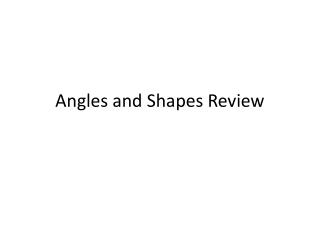DownloadDownload PresentationAngles and Shapes Review

# Angles and Shapes Review

Download Presentation## Angles and Shapes Review

- - - - - - - - - - - - - - - - - - - - - - - - - - - E N D - - - - - - - - - - - - - - - - - - - - - - - - - - -
##### Presentation Transcript

1. Angles and Shapes Review

2. Points, Lines, Angles C G A E U T H B F I D X J L Point ____________ Line Segment Line Intersecting Line Perpendicular Line Parallel Lines Ray Vertex Acute Angle Obtuse Angle Right Angle Straight Angle P M O N V S EQ: Where do we see angles in everyday life?

3. Please find each of the following on the attached picture. Please write the symbol and letter for each so I know what you found! Point ____________ Line Segment ______________ Line _____________ Intersecting Lines ____________________________ Perpendicular Lines _______________ Parallel Lines ________________

4. B A C D

5. Can you name these angles? 1 2 3 4

6. Polygon Graphic Organizer Equilateral Triangle Isosceles Triangle Scalene Triangle Right Triangle Obtuse Triangle Acute Triangle Rectangle Trapezoid Square

7. Polygon Graphic Organizer Parallelogram Rhombus Pentagon Hexagon Octagon Triangle Quadrilateral Irregular Polygon Regular Polygon

8. What is a polygon?

9. What is a polygon? When line segments are connected at endpoints to make a closed figure, a polygon is formed. Line segments are called SIDES.

10. What is a regular polygon? What is an irregular polygon?

11. What are regular and irregular polygons? A regular polygon has all sides equal lengths and all angles equal degrees. An irregular polygon does not have all sides equal length or all angles equal degrees.

12. How are polygons named?

14. What do I look like? I have only one pair of parallel sides.

15. I’m a trapezoid.

17. What do I look like? I have opposite sides parallel and opposite sides are the same length.

18. I’m a parallelogram.

20. What do I look like? My opposite sides are parallel and all four angles are right angles.

21. I’m a rectangle.

23. What do I look like? My opposite sides are parallel and my four sides are the same length.

24. I’m a rhombus.

26. What do I look like? I have four sides that are the same length and 4 right angles.

27. I’m a square.

29. What do I look like? I have 8 sides and 8 angles.

30. I’m an octagon.

31. What do I look like? I have six sides and six angles.

32. I am a hexagon.

33. What do I look like? I have 5 sides and 5 angles.

34. I’m a pentagon.

35. Properties of Triangles

36. What do I look like? I have 3 sides. None of my sides are the same length.

37. I’m a scalene triangle.

38. Properties of Triangles

39. What do I look like? I have 3 sides. At least 2 sides are the same length.

40. I’m an Isosceles Triangle

41. Properties of Triangles

42. What do I look like? I have 3 sides and all my sides are the same length and all my angles are the same.

43. I’m an equilateral triangle.

44. Properties of Triangles

45. What do I look like? I have 3 sides and one angle is a right angle.

46. I’m a right triangle.